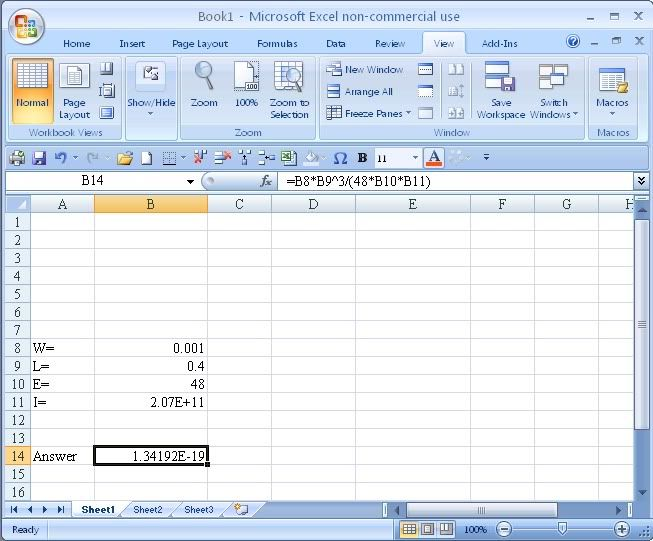1. I need some help with this formula
copy and past from Excel
steel actual deflection 0.15mm
0.001*0.4³/48*207000000000*5.316759994E-11=1.52mm

brass actual deflection 0.26mm
0.001*0.4³/48*105000000000*4.935823594E-11=7.18mm
0.002*0.4³/48*105000000000*4.935823594E-11= 1.43608E-05

I have to work out the theatrical deflection for steel and brass loads from 0.001 to 0.005N. My calculations for steel are close to actual, but for brass 0.001N load deflects more then 0.002N load. Calculation are done on excel as my calculator shows sentex error :?

Any help will be appreciated.2. ### Related Discussions:

• In excel you need to place parentheses around the denominator terms. Otherwise you are multiplying instead of dividing.• you mean the (number,power) thing? I removed it to make it easy to read the post• When you wrote "copy and paste" I foolishly assumed you meant "copy and paste".
What I mean is:

0.001*0.4³/48*207000000000*5.316759994E-11

should be written in Excel as:

0.001*0.4³/(48*207000000000*5.316759994E-11)

if everything after the slash is supposed to be in the denominator.• ok im new to excel

this is how I did it

=B8*POWER(0.4,3)/48*20700000000*C2• WL³/48EI

Let W be in cell B8,
Let L be in cell B9
Let E be in cell B10
Let I be in cell B11

then WL³/48EI should be written as:

=B8*B9^3/(48*B10*B11)• Does this help?•Originally Posted by Wild CobraDoes this help?yes, only after your answer confused me, your figure for I is for E, I=5.32E-11.

Thank you Wild Cobra and Bunbury.

Just one more question, would I write 1.21E-07 as 0.121x10ˉ7? the actual deflection was 0.15mm• Exponential sign in excel is **, just like Fortran, hence U shld write

0.001*0.4**3/(48*207000000000*5.316759994E-11)Originally Posted by BunburyWhen you wrote "copy and paste" I foolishly assumed you meant "copy and paste".
What I mean is:

0.001*0.4³/48*207000000000*5.316759994E-11

should be written in Excel as:

0.001*0.4³/(48*207000000000*5.316759994E-11)

if everything after the slash is supposed to be in the denominator.• Interesting. As I recall the original post had the caret ^ to indicate exponentiation, but the forum software seems to have made it a superscript. The ^ does work in Excel. Maybe ** does too, I never tried it.• Maybe ** does too, I never tried it
It doesn't work in Excel 2003. ** is the symbol for x 10^4 but it's quicker to write e:

3^4 = 81
3**4 = 30000
3e4 = 30000Bookmarks
 Posting Permissions
 You may not post new threads You may not post replies You may not post attachments You may not edit your posts   BB code is On Smilies are On [IMG] code is On [VIDEO] code is On HTML code is Off Trackbacks are Off Pingbacks are Off Refbacks are On Terms of Use Agreement